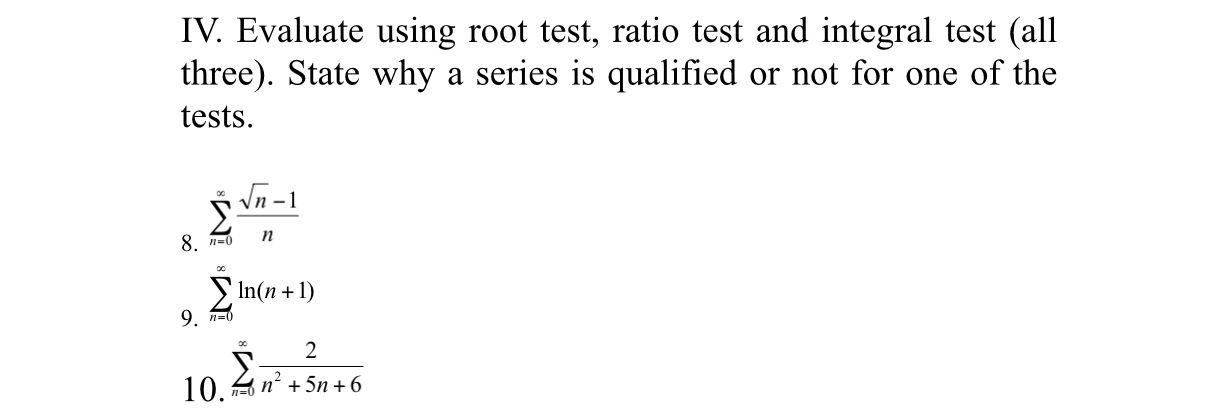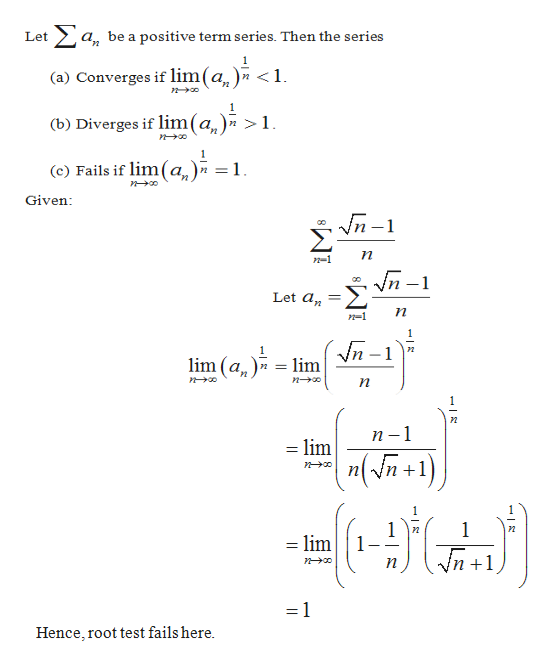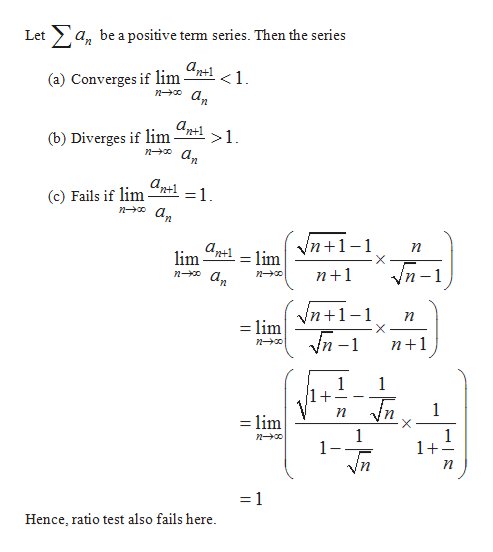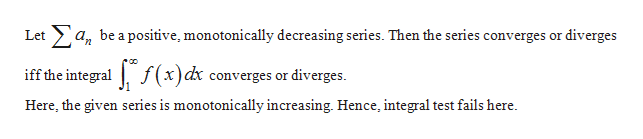# IV. Evaluate using root test, ratio test and integral test (allthree). State why a series is qualified or not for one of thetestsVn-1п8 п-0In(n9. п-0210.Tn5n6+

Question
1 viewshelp_outlineImage TranscriptioncloseIV. Evaluate using root test, ratio test and integral test (all three). State why a series is qualified or not for one of the tests Vn-1 п 8 п-0 In(n 9. п-0 2 10. Tn5n6 + fullscreen
check_circle

Step 1

(Note: We’ll answer the first question since the exact one wasn’t specified. Please submit a new question specifying the one you’d like answered)

Root test is the test for the positive term series. It states that,help_outlineImage TranscriptioncloseLeta, be a positive term series. Then the series (a) Converges if lim(a )» <1. (b) Diverges if lim(a,) > n 1. (c) Fails if lim(a)n = 1 Given Vn- Ση. п n-1 л -1 Let a п n-1 Vn-1 lim 2 lim (a п п-1 lim "(/n+1) n 1 lim 1 1 п = 1 Hence, root test fails here. fullscreen
Step 2

Ratio test for positive term series:help_outlineImage TranscriptioncloseLeta, be a positive term series. Then the series аp1 <1. (a) Converges if lim а, (b) Diverges if lim = 1 (c) Fails if lim noa Vn1 X аp1 lim п = lim n-o an n+1 п -1 Vn1- X п lim n+1 1 1+ 1 1 = lim X 1 1 1 1+ no n п = 1 Hence, ratio test also fails here fullscreen
Step 3

Integral te...help_outlineImage TranscriptioncloseLeta bea positive, monotonically decreasing series. Then the series converges or diverges iff the integral f(x)dx converges or diverges. Here, the given series is monotonically increasing. Hence, integral test fails here. fullscreen

### Want to see the full answer?

See Solution

#### Want to see this answer and more?

Solutions are written by subject experts who are available 24/7. Questions are typically answered within 1 hour.*

See Solution
*Response times may vary by subject and question.
Tagged in